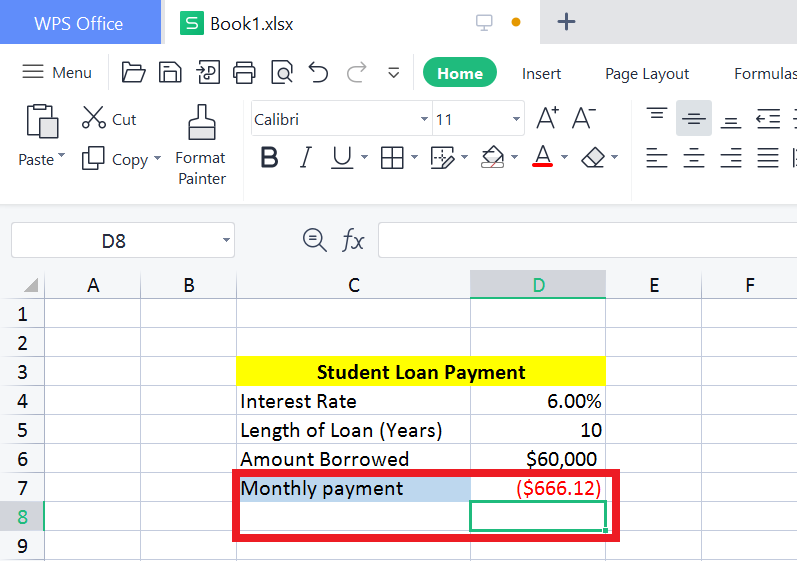# How to Calculate Monthly Payments in Excel Using Formulas

July 22, 2022
1.8K Views
0

A free Office suite fully compatible with Microsoft Office

A free Office suite fully compatible with Microsoft Office

Every sector now a day, waiting to avail the opportunity to use this smart tool through which they can easily prepare and maintain financial statements on monthly/ semi-annually/ yearly basis. Using this software, also help you to look after the loan payments and many more.

There are many other ways to calculate the loan/credits, mortgages, students loan, their payments & all. But the best way to have the payment check is through a function method.

Calculate the monthly payment through Formulas: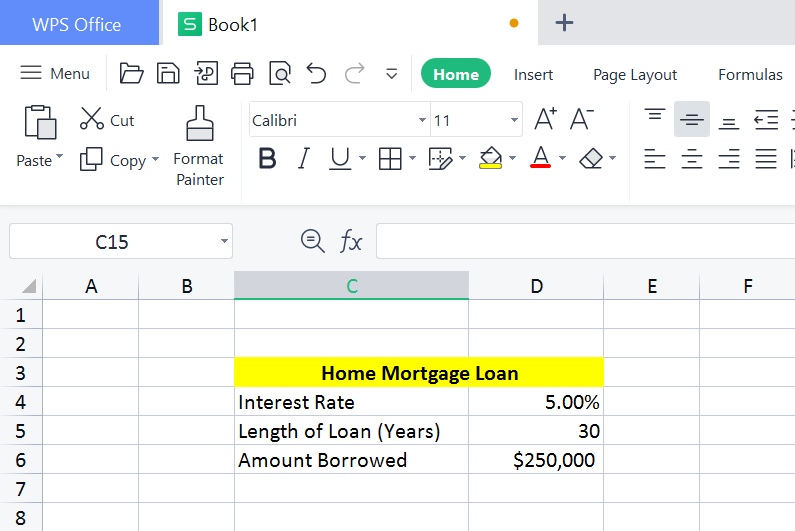If you’re having a mortgage, and you want to know the total predicted amount of expected time. Just for an example, we took three information to calculate what we have within our predicted time; all of the details are listed below:

Interest: 5%

Loan limit (years): 30 years

Total credit amount: \$250,000

Once you have this information, then on the formula bar you have to write down “=PMT (“. It will show the suggestive formula through which you can enter all the numbers according to the data. (1 Year = 12 Months).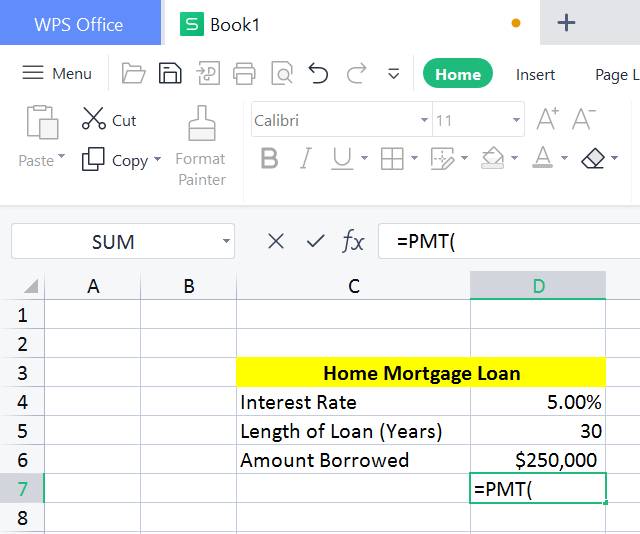Steps:

1.But we will divide the interest rate with 122.Multiply the loan limit with 12.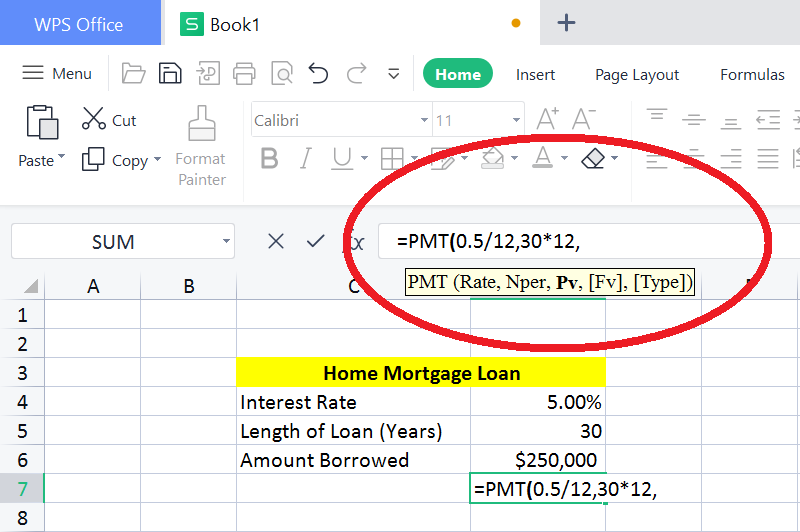3.It will be written in the formula bar as =PMT(0.5/12,30*12,250,000)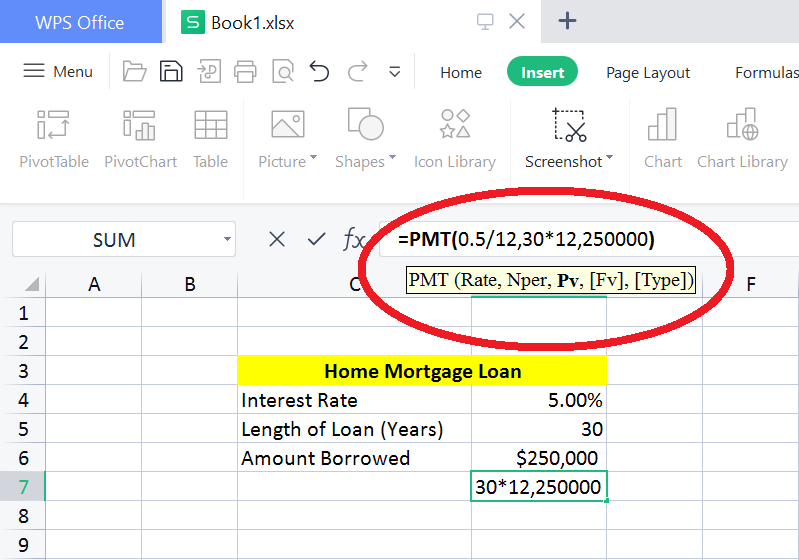1. The answer will be mentioned as monthly payment.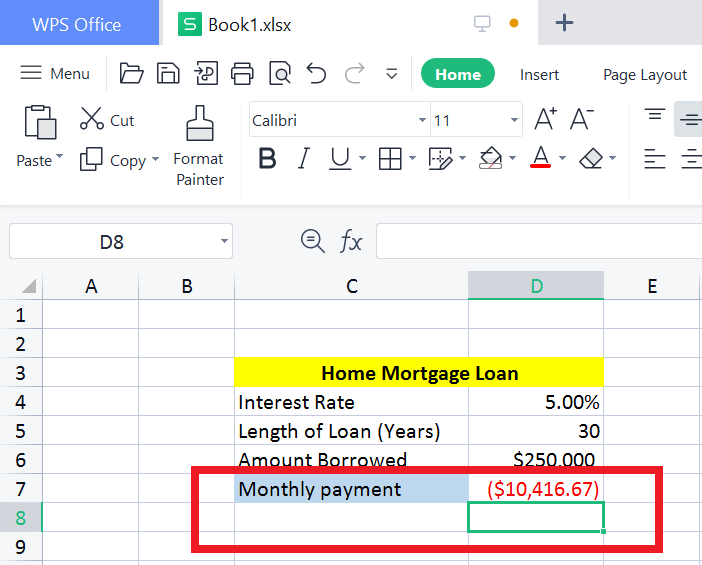Calculating the Monthly Car Payment in Excel:

When we calculate the car loan its similar to the mortgage calculation. Let’s take an example; we have a mortgage payment of interest rate of 4%, with the total 6 years as loan limit and the borrowed amount is \$30,000. Preparing the data accordingly:

Interest Rate: 4.00%

Loan limit: 6 years

Amount credited: \$30,000Steps:

1. The interest rate as D4(as it is written in column 4 but row is D) & we will divide the 12 with interest rate.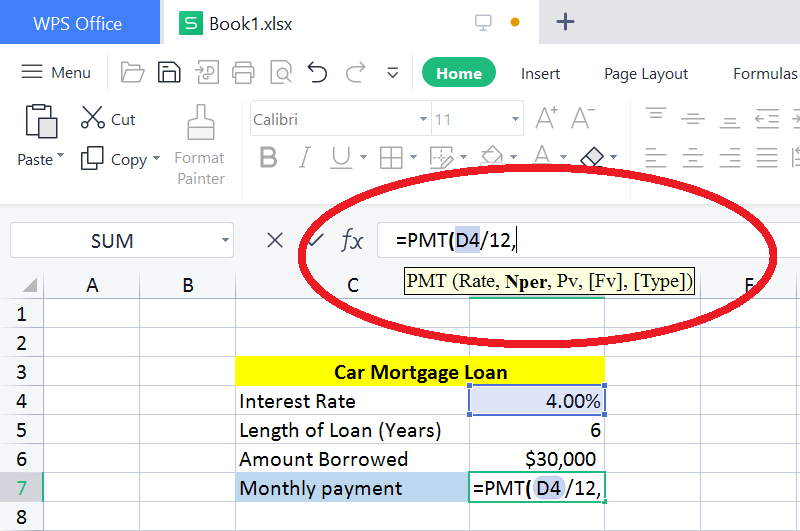1. Multiply 12 with loan limit.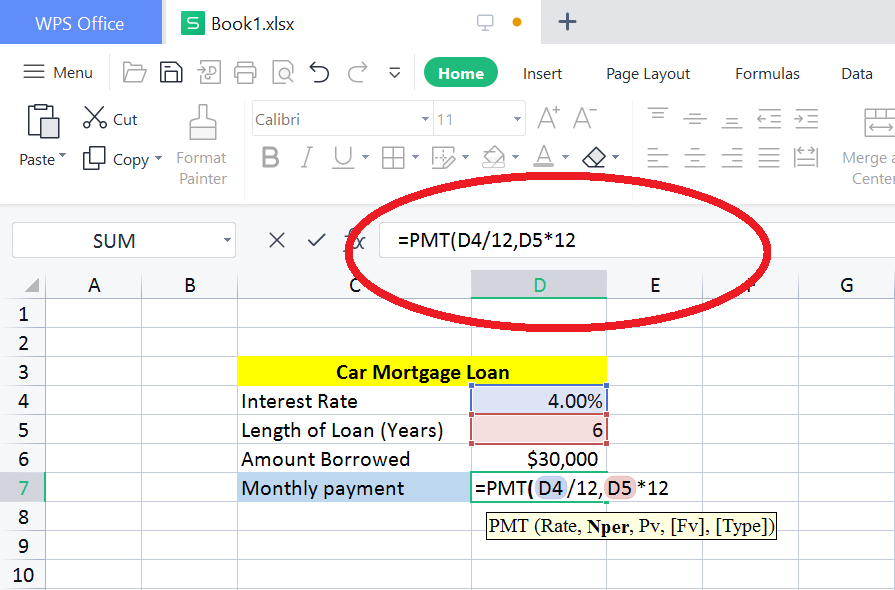1. Now, we will mention the total amount borrowed i.e. \$30,000, Same as the above, in the formula bar we will mention, =PMT(D4/12,D5*12,30,000)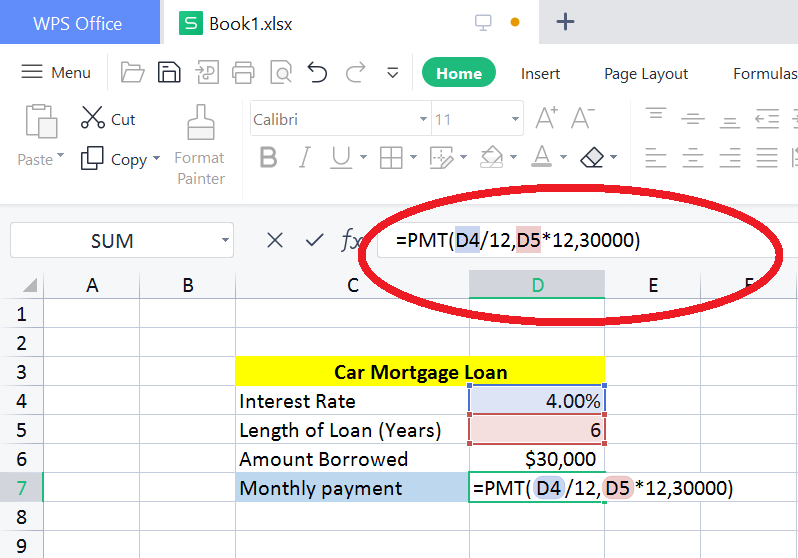1. In the next row you will mention the answer as the monthly payment.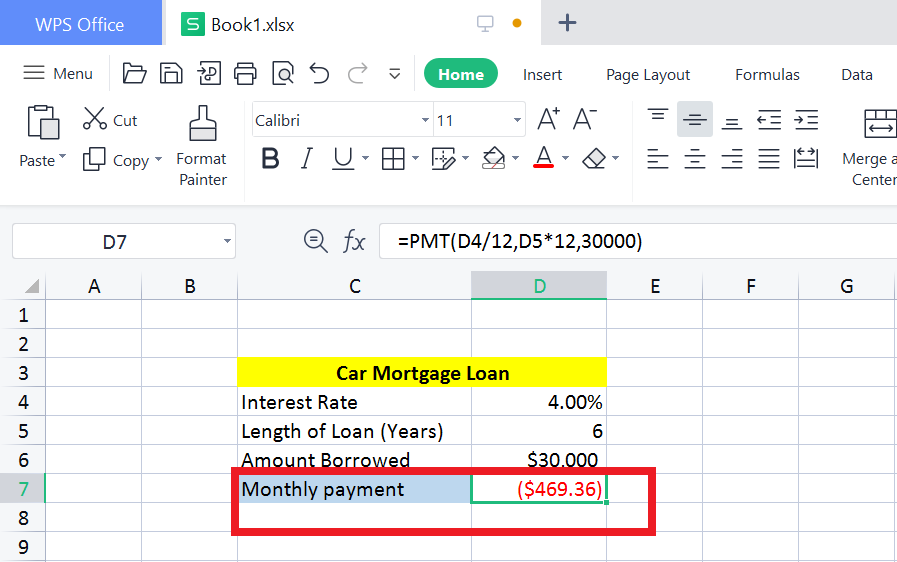Calculating the Student Monthly Loan Payments in Excel:

Just like the car mortgage loan payment, we have a student monthly loan payment where we can easily find out the future value of the current payment. We have interest rate of 6% with 10 years, length limit of loans and the total borrowed amount is \$60,000. We can easily find out the loan payments through the excel.

Interest rate: 6.00%

Length limit of loans: 10 years

Total credited amount: \$60,000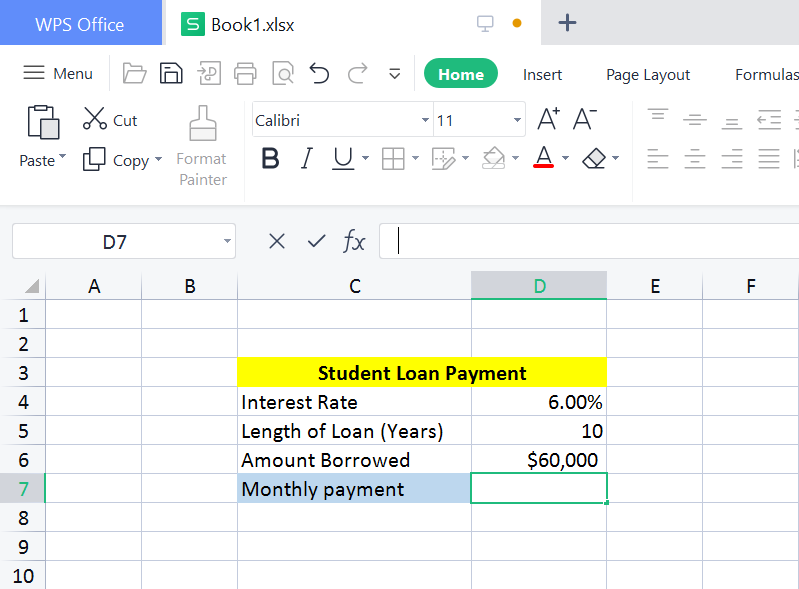STEPS:

1. Start the formula with =PMT(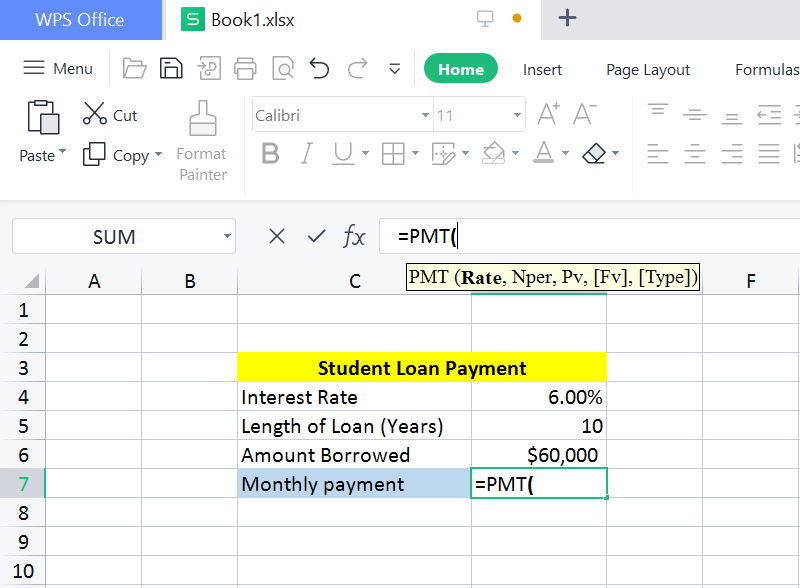1. enter the interest rate as D4, divide with 12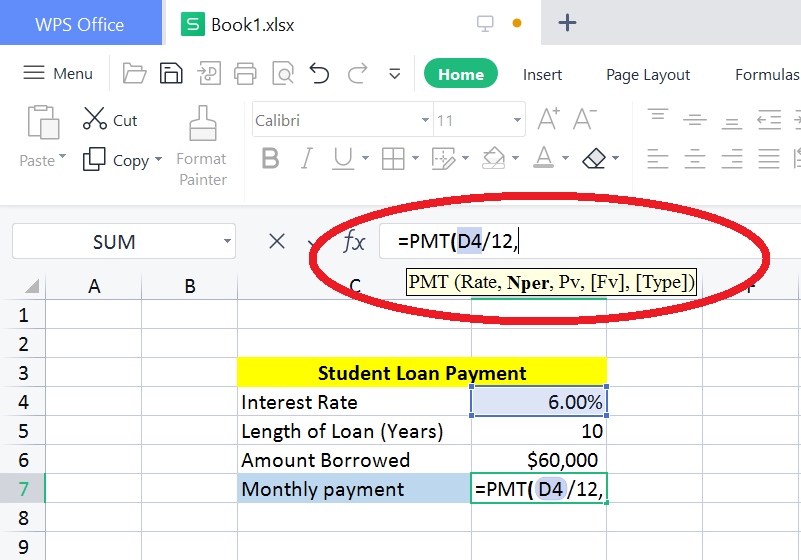1. enter the total years as D5, and multiply with 12.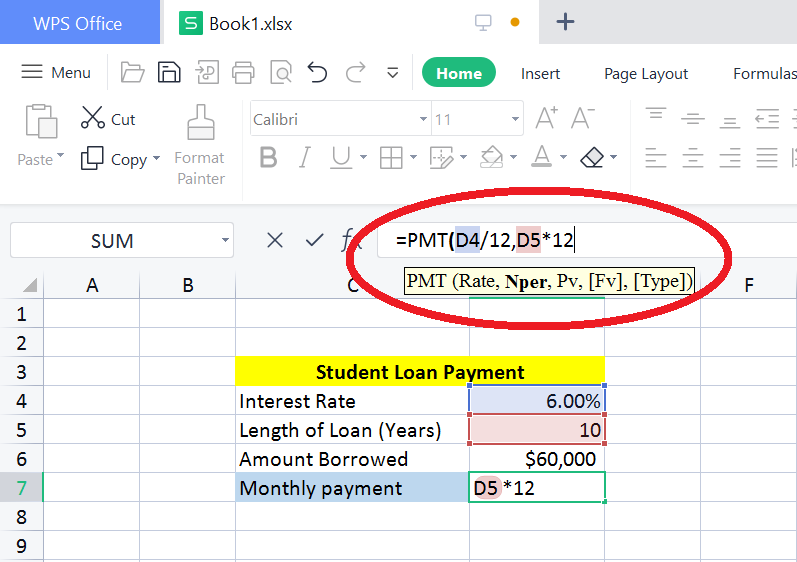1. Enter the total borrowed amount as D6.

1. Mention down the total monthly payment.